# Condense Logarithms Calculator

By Maciej Kowalski, PhD candidate
Last updated: Feb 08, 2021

Welcome to Omni's condense logarithms calculator, where we'll see how to rewrite logarithms or rather logarithmic expressions as a single logarithm. To be precise, we'll try simplifying logs by applying three simple formulas. In fact, we'll use the same ones that work for expanding logarithms; we'll simply do it all backward. As such, we'll find out how to subtract and how to add logs (with the same base, mind you).

## What is a logarithm?

With the COVID-19 pandemic raging on, there's been quite some talk about the rate at which the number of cases increases over time. Mathematically speaking, such a thing is called exponential growth.

As you might have noticed, the name suggests that it has something to do with exponents. Indeed, if we assume that each infected person transmits the disease onto, say, 4 others, then patient zero got 4 people sick. In turn, they got 4² = 4 * 4 = 16 people infected, who later got 4³ = 4 * 4 * 4 = 64 people infected. In general, we describe the number of sick in the n-th step by the exponent 4ⁿ.

The logarithm is the inverse function to the exponential one. To make it all precise, let's see the following log definition.

 💡 logₐ(b) gives you the power to which you'd need to raise a in order to obtain b. Note, however, that in general, this can be [a fractional exponent](calc:1503).

In the above epidemic example, the logarithm (with base 4) returns at which step we get a fixed number of infected. For instance:

log₄64 = 3

Note that taking a root is also considered an inverse operation to taking a power. However, as opposed to logarithms, roots return the exponent base, not the exponent itself (in the above language: they return how many people get infected by a single person). For example:

³√64 = 4

Before we learn how to rewrite logs, let's mention a few critical facts concerning them.

• There are two very special cases of the logarithm which have unique notation: the natural logarithm and the logarithm with base 10. We denote them ln(x) and log(x) (the second one simply without the small 10), and their bases are, respectively, the Euler number e and (surprise, surprise!) the number 10. There is also the binary logarithm, i.e., log with base 2, but it's not as common as the first two.
• The logarithm function is defined only for positive numbers. In other words, whenever we write logₐ(b), we require b to be positive.
• Whatever the base, the logarithm of 1 is equal to 0. After all, whatever we raise to power 0, we get 1.
• Logarithms are extremely important. And we mean EXTREMELY important. Outside of mathematics, they're used in statistics (e.g., the lognormal distribution), economics (e.g., the GDP index), medicine (e.g., the QUICKI index), and chemistry (e.g., the half-life decay). Also, quite a few physical units are based on logarithms, for instance, the Richter scale, the pH scale, and the dB scale.

Alright, that should be enough of a description for now. It's time to get back to mathematics and try simplifying logs using concrete formulas. We'll take on some gruesome expressions that involve logs and learn to write the expressions as a single logarithm.

## How to rewrite logarithms?

As we've mentioned in the first section, logarithms are the inverse operation to exponents. Therefore, it should come as no surprise that the properties of the two are quite connected: in particular to multiplying and dividing exponents.

Let us begin by mentioning one log property that our condense logarithms calculator doesn't use - the change of base formula. It allows us to switch from one log basis to another, but at a price - we get a quotient of two expressions from a single one. If you'd like to see the specifics, make sure to check out Omni's dedicated change of base calculator.

We will, however, use here three other formulas. We often use them for expanding logarithms, but there's no harm in working them the other way round: for condensing logs instead. Still, let us see them in their original form.

• The logarithm of a product is a sum of logarithms.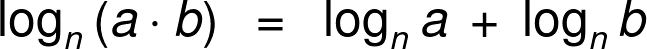• The logarithm of a quotient is a difference of logarithms.• The logarithm of an exponent is a multiple of a logarithm.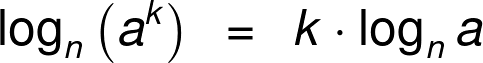As you can see, all of them take a single log (of a product, quotient, or exponent) and expand it into a longer expression. However, if we look at them backward, they write the expression as a single logarithm. That way, we obtain formulas for adding logs, subtracting logs, and multiplying logs by a number. The three combined are what the condense logarithms calculator is all about.

## How to condense logarithms?

We now take the theory on how to rewrite logarithms and work its magic to our needs. Mind you, we'll keep to formulas for now, and once we have them in full glory, we'll move on to condensing logs examples in the last section.

First of all, we take on the simplest of the expanding formulas: that for a logarithm of an exponent. Let's turn it around, fix the notation to suit the one used in the condense logarithms calculator, and have it neatly here for future use: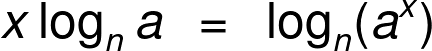We'll now use it (together with the product property) to learn how to add logs. And we don't mean just any sum - we mean adding logs with multiples.

x * logₙ(a) + y * logₙ(b) = ?

We begin by applying the first condensing logs formula to both summands, i.e., we take x and y and drag them inside:

x * logₙ(a) + y * logₙ(b) = logₙ(aˣ) + logₙ(bʸ).

Now, with no multiples in front of the expressions, we're able to go from adding logs to a log of a product: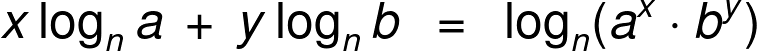Well, we've seen how to add logs, so it shouldn't be too difficult to go from there to subtracting logs, right?

x * logₙ(a) - y * logₙ(b) = ?

Indeed, it isn't. We start the same: by dragging x and y inside,

x * logₙ(a) - y * logₙ(b) = logₙ(aˣ) - logₙ(bʸ),

and we finish by translating subtracting logs into a log of a quotient.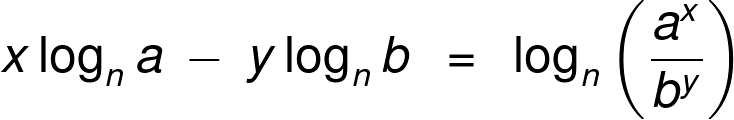Voilà! These three are all the formulas we'll need for simplifying logs. So let's now switch from symbols to numbers and get an example going with the condense logarithms calculator at hand.

## Example: using the condense logarithms calculator

With all the explanations we've seen so far, there's no need for any introduction - let's simply work out an example. Although technically, we've just done an introduction, haven't we? Oh, bother...

We'll show how to condense the logarithms in:

3 * log₆4 + log₆9

Before we write the expression as a single logarithm ourselves, let's see how to rewrite the logs with the condense logarithms calculator.

At the top of our tool, we choose the type of operation we're dealing with. In our case, we have a sum, so we choose "adding logs" under "Use the formula for." That shows us a symbolic expression with the notation used underneath: x * logₙ(a) + y * logₙ(b). Comparing it to ours, we see that we need to input:

x = 3, a = 4, b = 9, and n = 6.

However, this still leaves y. Recall that by convention, no number in front of a function (in this case, a logarithm, but it's the same for, say, trigonometric functions) means that the number is, in fact, equal to 1. Nevertheless, we don't need to input y = 1 since the condense logarithms calculator will understand a blank field as y = 1.

Once we give all the necessary data, the tool will spit out the answer underneath together with a step-by-step application of the formulas from the above section and a numerical approximation of the result. However, before we reveal it to the world, let's describe how to add the logs ourselves.

Firstly, just as we did in the above section, we drag the 3 from in front of the log inside using the exponent property:

3 * log₆4 + log₆9 = log₆(4³) + log₆9 = log₆64 + log₆9.

Next, we use the formula for how to add logs and get

3 * log₆4 + log₆9 = log₆64 + log₆9 = log₆(64 * 9) = log₆576 ≈ 3.54741.

In general, logarithms can be challenging to compute, so we get approximations like the one above with external tools - something like the condense logarithms calculator. Or any of Omni's algebra calculators - be sure to check them out!

Maciej Kowalski, PhD candidateUse the formula for
Variables
x
a
y
b
n
People also viewed…

### Books vs e-Books